Question

# Problem 2: Figure shows a pump and pipe network being used to transport heptane at 80F...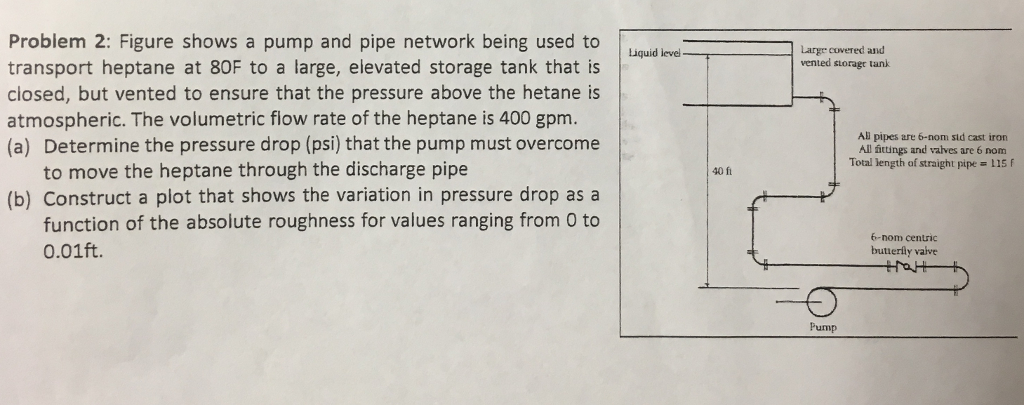Problem 2: Figure shows a pump and pipe network being used to transport heptane at 80F to a large, elevated storage tank that is closed, but vented to ensure that the pressure above the hetane is atmospheric. The volumetric fiow rate of the heptane is 400 gpm. (a) Determine the pressure drop (psi) that the pump must overcome Liquid level Large covered and vented storagt tank Al pipes are 6-nom sid cast iron Al fattings and valves are 6 nom Total length of straight pipe 115 to move the heptane through the discharge pipe 40 fi (b) Construct a plot that shows the variation in pressure drop as a function of the absolute roughness for values ranging from O to 0.01ft. b-nom centric butterfly valve Pump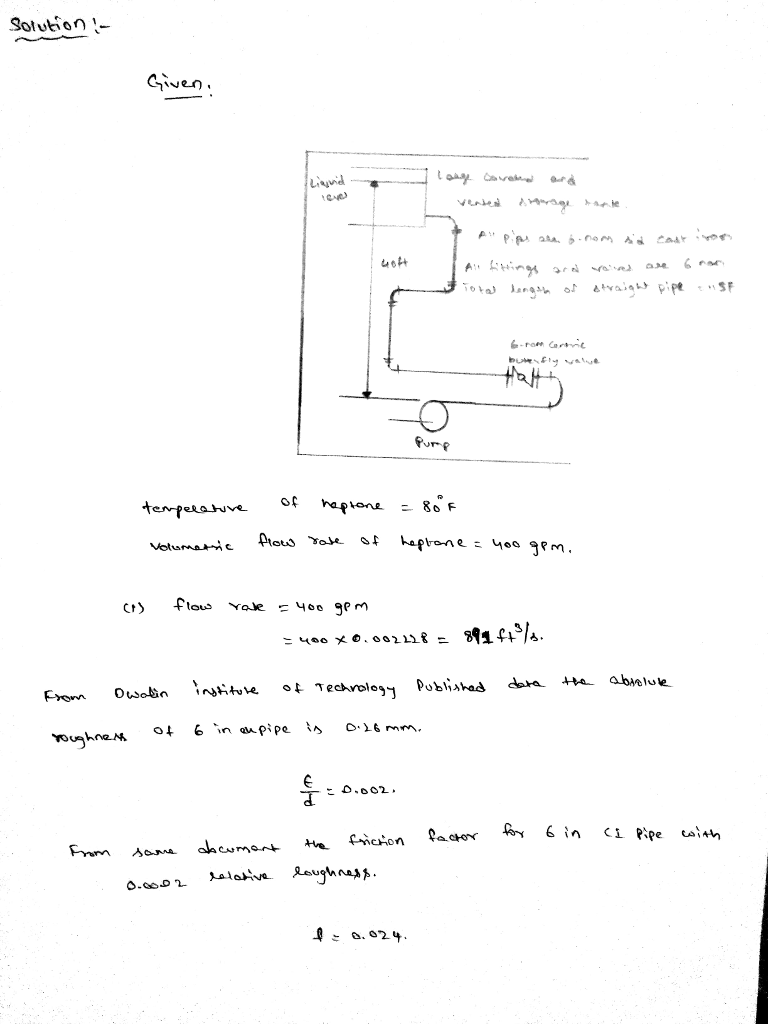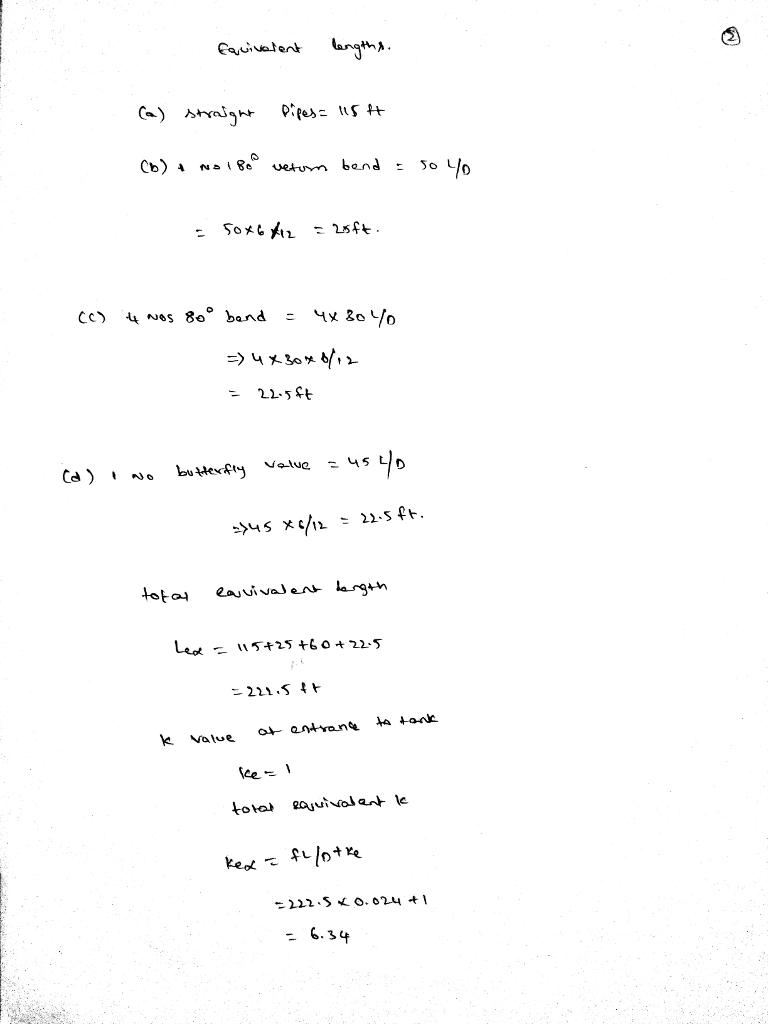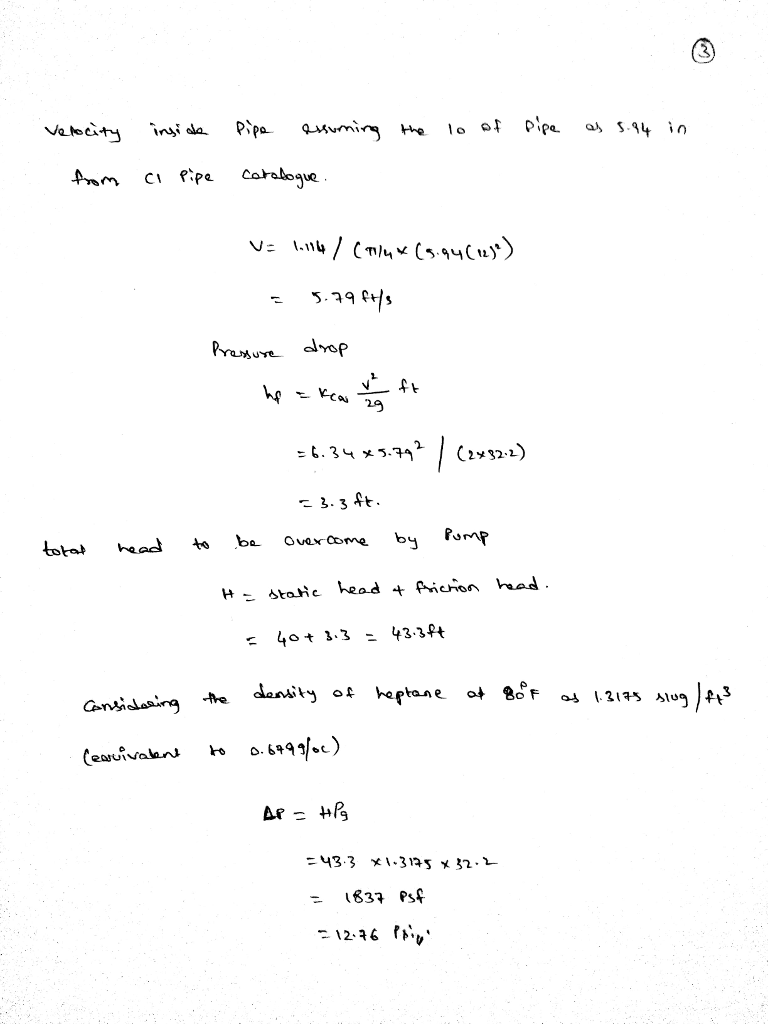#### Earn Coins

Coins can be redeemed for fabulous gifts.

Similar Homework Help Questions
• ### The system below shows a simplified schematic of an oil transfer system between tanks, from left...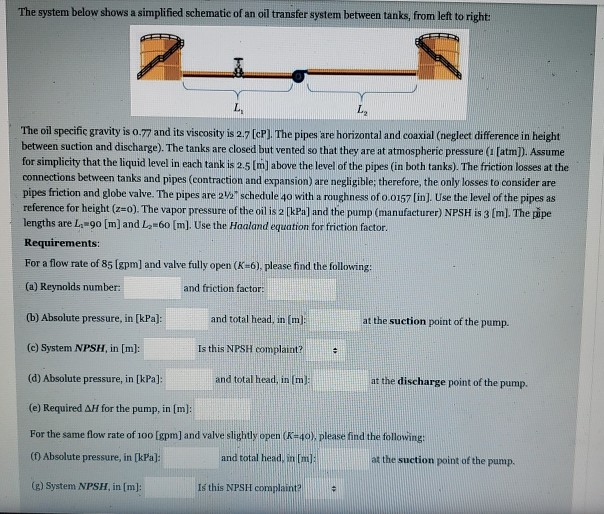The system below shows a simplified schematic of an oil transfer system between tanks, from left to right: L L The oil specific gravity is 0.77 and its viscosity is 2.7 [cP). The pipes are horizontal and coaxial (neglect difference in height between suction and discharge). The tanks are closed but vented so that they are at atmospheric pressure ([atm]). Assume for simplicity that the liquid level in each tank is 2.5 (m) above the level of the pipes (in...

• ### Problem #5 The figure below shows a submersible pump being used to circulate 60L/min of water...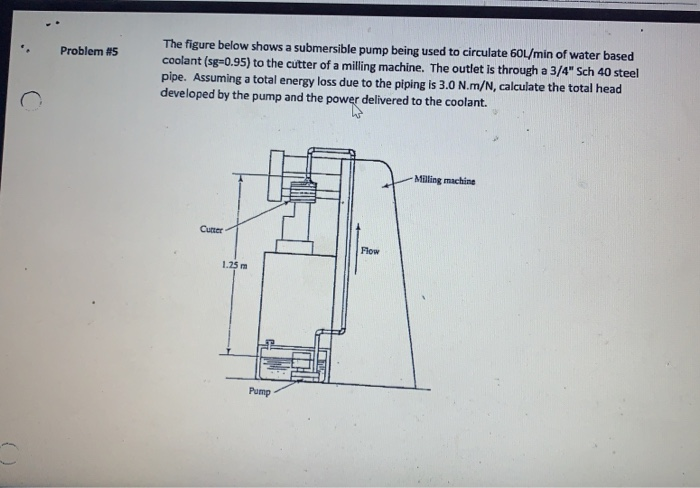Problem #5 The figure below shows a submersible pump being used to circulate 60L/min of water based coolant (sg=0.95) to the cutter of a milling machine. The outlet is through a 3/4" Sch 40 steel pipe. Assuming a total energy loss due to the piping is 3.0 N.m/N, calculate the total head developed by the pump and the power delivered to the coolant. Milling machine Flow . C Problem 14 The pipeling shown takes water from a reservoir and terminates...